# DAY Function

Returns the day number of a date

## What is the DAY Function?

The DAY Function is categorized under Excel Date/Time Function. In financial modeling, we often need to analyze time-series data such as revenue. For example, in a restaurant business, we might be interested in knowing which day of the week is the busiest or in finding out if there is a trend emerging. For a fashion boutique, we may want to see which day of the month has the most customers coming in. We may be interested in knowing which days when most purchase orders are placed or shipments are done.

The DAY Function is useful for financial analysts as it returns the day of a date, represented by a serial number. The day is given as an integer ranging from 1 to 31.

### Formula

=DAY(Serial_number)

Where:

Serial_number (required argument) – This is the date of the day we are trying to find. We need to provide the dates to the DAY function as:

• Serial numbers
• Date values returned from other Excel functions
• Reference to cells containing dates

### How to Use the DAY Function in Excel?

To understand the uses of the DAY function, let’s consider a few examples:

#### Example 1

Suppose we are given the following dates. Let’s see how the function returns a result.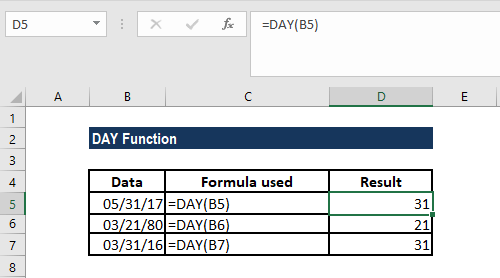In the example above:

• We provided the dates supplied as references to cells containing dates or (in the example in cell A2) as a date returned from another Excel function.
• Often, the result you get would look like a date such as 1/1/1900, instead of an integer. This arises due to the cell or column being formatted as a “date”. Remember here that the only issue is the formatting of the cell and not the value returned by the function. To correct this, we need to select the “General” formatting option from the drop-down format menu of the Excel ribbon. It is located in the “Number” group, on the Home tab of the ribbon, as shown below: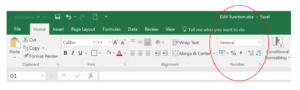#### Example 2

Let’s assume we need to add a given number of years to dates. In such a scenario, this function is useful. The data given is: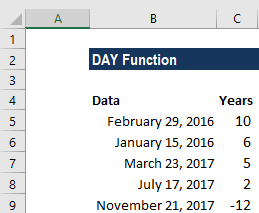The formula to use is: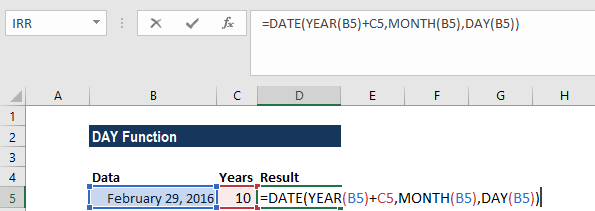We get the following result: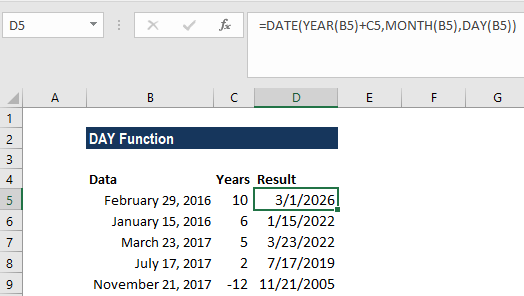Here, in the given formula, the YEAR, MONTH, and DAY functions extract those respective date components. For A2:

= Year 2016

= Month 2 (Feb)

= Date 29th

The DATE function helps in reassembling the component values back into a valid Excel date. In order to add years or to subtract years from the given date, we just need to add the value in B2 to the year component prior to the reassembly, so the formula we used is =DATE(YEAR(A2)+B2,MONTH(A2),DAY(A2)).

#### Example 3

Suppose we wish to get the first day of the month for a given date. For this, we can form and use a simple formula based on the DAY function.

The data given is :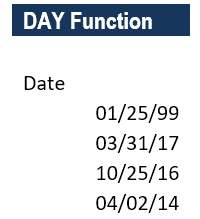The formula used is =B5-DAY(B5)+1, as shown below: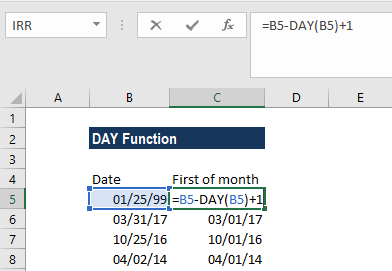We get the following result: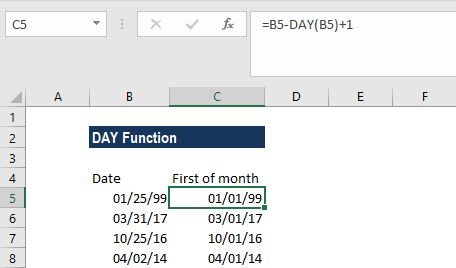Let’s now understand how this function works. Here, when we used the DAY function, it would return the day value for a date. As shown in the example, the day value for the date in cell A2 (January 25, 1999) is 25. Remember that dates are stored in Excel as serial numbers. As the dates are just serial numbers in Excel, we can subtract 25 and then add 1 to get the date value for January 1, 1999.

Thanks for reading CFI’s guide to important Excel functions! By taking the time to learn and master these functions, you’ll significantly speed up your financial analysis. To learn more, check out these additional CFI resources:

• Excel Functions for Finance